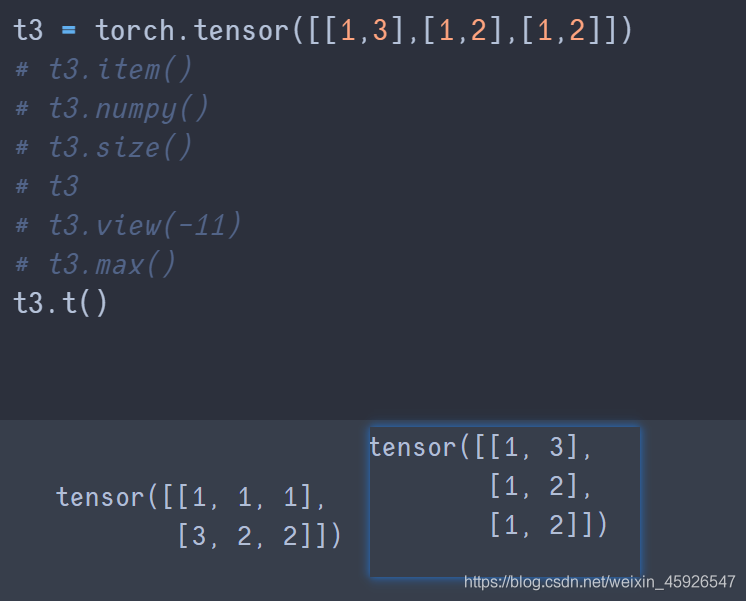# Python深度學習之Pytorch初步使用

## 一、Tensor

Tensor(張量是一個統稱，其中包括很多類型)：

0階張量：標量、常數、0-D Tensor；1階張量：向量、1-D Tensor；2階張量：矩陣、2-D Tensor；……

## 二、Pytorch如何創建張量

### 2.1 創建張量

```import torch

t = torch.Tensor([1, 2, 3])
print(t)```### 2.2 tensor與ndarray的關系

```import torch
import numpy as np

t1 = np.array(torch.Tensor([[1, 2, 3],
[1, 2, 3]]))
t2 = torch.Tensor(np.array([[1, 2, 3],
[1, 2, 3]]))
```### 2.3 常用api

torch.empty(x,y)torch.ones([x, y])torch.zeros([x,y])zeros與empty的區別

torch.rand(x, y)torch.randint(low, high, size)torch.randn([x, y])

### 2.4 常用方法

item()numpy()size()view()

`淺拷貝`，tensor的`形狀改變`dim()max()t()transpose(x,y)

x，y是size裡面返回的形狀相換。permute()transpose與permute的區別## 三、數據類型### 3.1 獲取數據類型

tensor.dtypetype()

`修改`數據類型。## 四、tensor的其他操作

### 4.1 相加### 4.2 tensor與數字的操作

`tensor + 數值`## 五、CUDA中的tensor

`CUDA` (Compute Unified Device Architecture)，是NVIDIA推出的運算平臺。CUDATM是一種由NVIDIA推出的通用並行計算架構，該架構使GPU能夠解決復雜的計算問題。
torch.cuda這個模塊增加瞭對CUDA tensor的支持，能夠在cpu和gpu上使用相同的方法操作tensor通過`.to`方法能夠把一個tensor轉移到另外一個設備(比如從CPU轉到GPU)